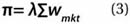# Efficient Income Investing

December 21, 2012

To conform to standard practice, we use forward estimates of capital market returns. Predicting returns is a difficult exercise and can result in portfolios lacking in intuitive appeal and economic value. For example, Chopra and Ziemba (1993) estimate that at moderate risk tolerance levels, minimum variance optimisation is 11 times more sensitive to estimation error of returns than to estimation error in the covariance matrix. Our returns are based on the Sharpe-Lintner-Mossin-Treynor Capital Asset Pricing Model (CAPM). More specifically, we use the reverse optimisation procedure from Sharpe , which is also the starting point for the Black-Litterman model (see Black and Litterman ). The process is described in equation 3Where:

• Π = an N vector of excess returns
λ = the risk aversion coefficient
= an N by N covariance matrix of excess returns
wmkt = an N vector of the market capitalization weights (the CAPM market portfolio)

Market capitalisation data are used to estimate the CAPM market portfolio. For most of our asset classes, we use index weights as a proxy for market weights. The market capitalisation for equities is based on data from the World Bank and market capitalisation for fixed income is based on data from the Bank for International Settlements.

The market capitalisation values for real estate are based on an approach used in Idzorek, Barad, and Meier , using J.P. Morgan Asset Management Real Estate’s estimate of the value of direct US real estate investment

The covariance matrix is constructed using monthly returns from January 1993 to April 2012. The assumed risk-free rate is 3.83 percent2 and the market risk premium is 4.35 percent, which was solved through reverse optimisation to create a large-cap domestic equity premium of 6.35 percent Relative market capitalisation weights and the resulting return estimates are included in Figure 5.

The values in Figure 5 are assumed to be the total return values for our analysis. We then split this total return into price and income returns. For fixed income, we assume that 100 percent of the total return comes from income and for equities we use asset class history to determine the income and price return split. For TIPS, which have their principal adjusted, we do the same.For equities, price appreciation is going to drive the majority of the return, except for preferred stocks, where the entire total return is assumed to be income return. With respect to dividends, yields are estimated based on recent dividend yields for the respective equity indices as well as long-term general historical trends. Covariances are calculated from the historical returns. The average return and standard deviation for the income and price return components of the total return are included in Figure 6. While the total return will always be the sum of the income return and price return, the standard deviations are not additive.

Figure 6 provides information on the how the relative efficiency of a portfolio (using minimum variance criteria) could vary, depending upon the investor’s underlying goal. For example, an investor entirely focused on income would likely consider long-dated government bonds an attractive investment since they offer a relatively high level of income return with a low standard deviation of income; however, they also have significant price return volatility. An investor purchasing a bond directly and planning to hold it to maturity would effectively experience no volatility. However, we assume the investor doesn’t have a fixed horizon and is thus sensitive to price movements.

For a larger view, please click on the image above.